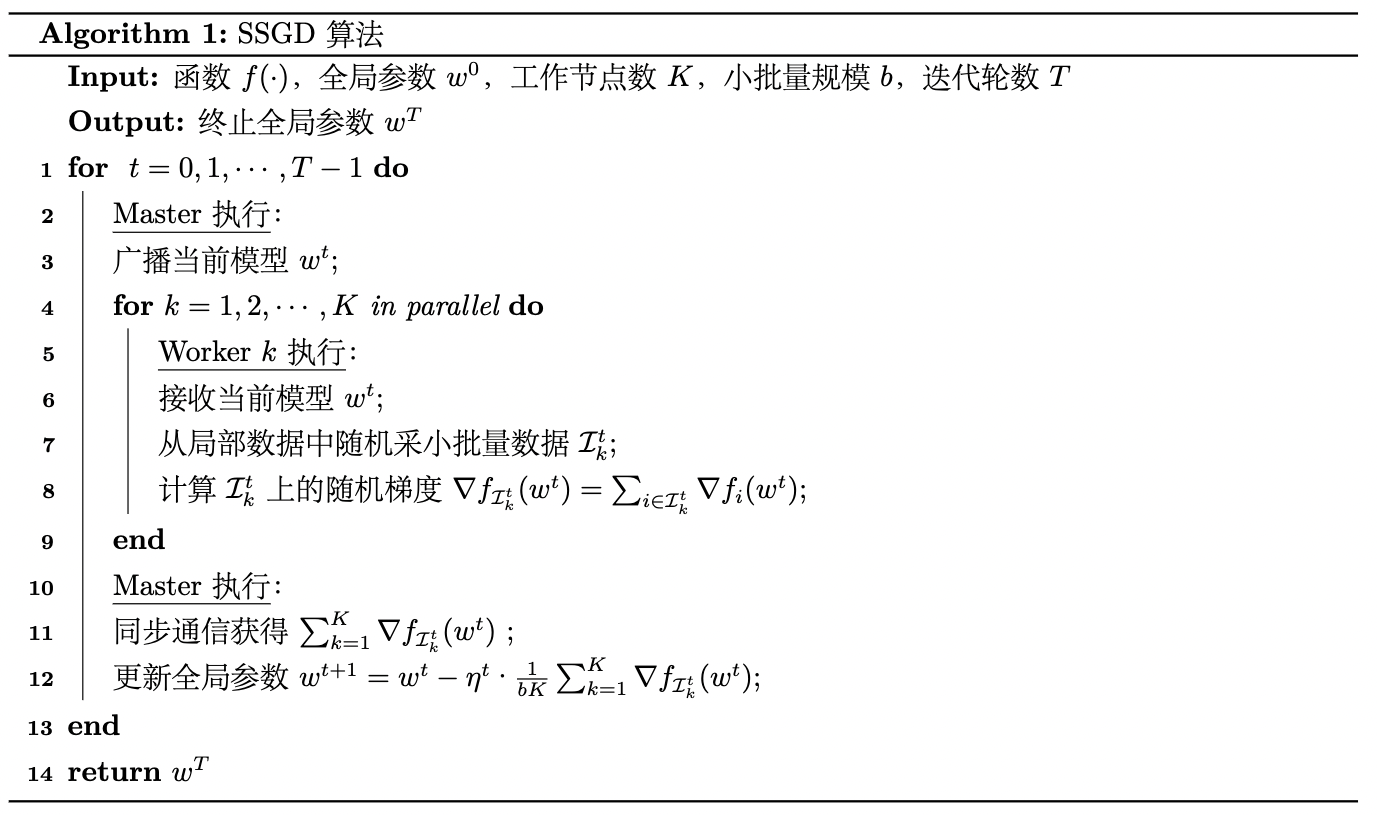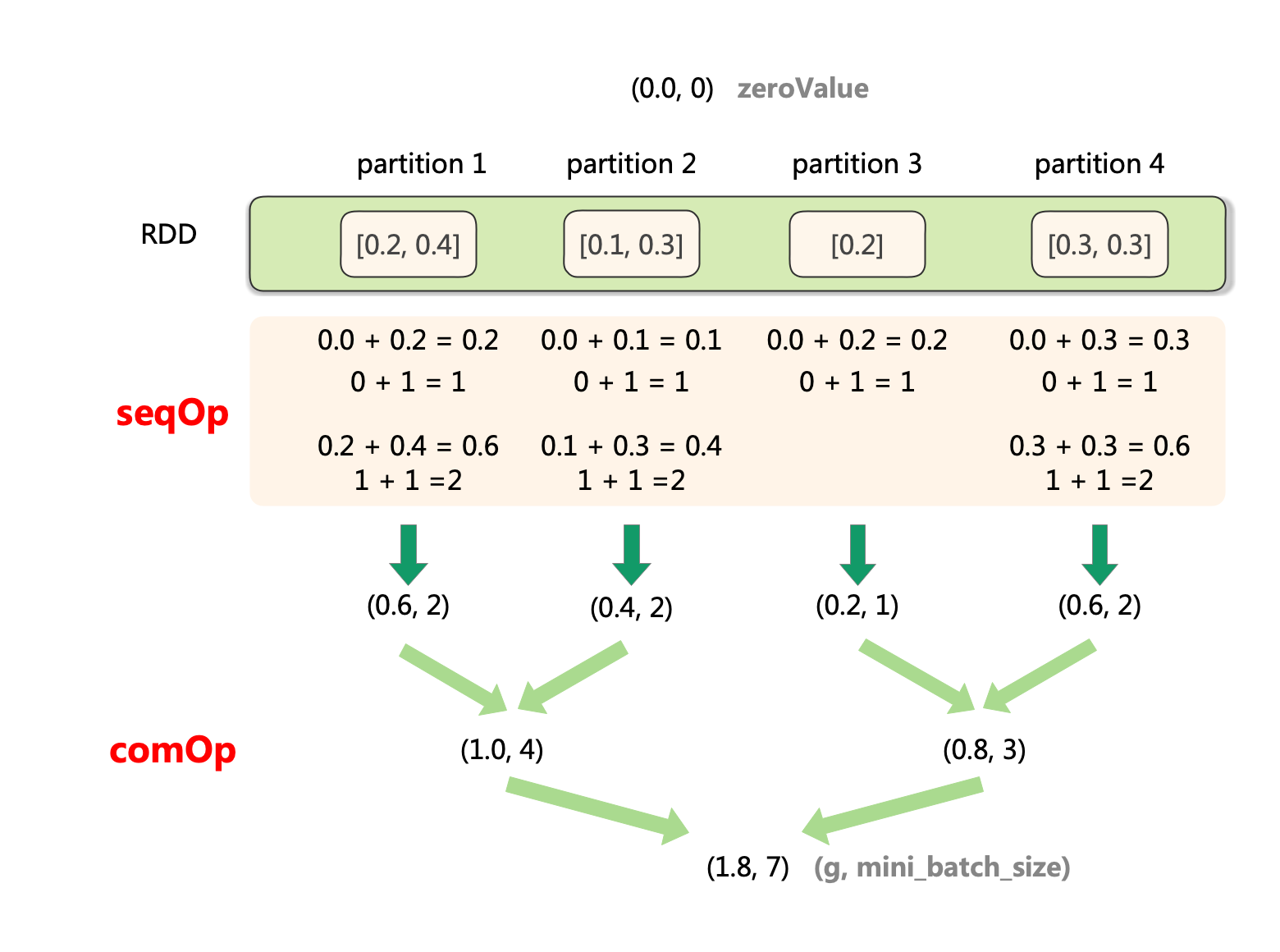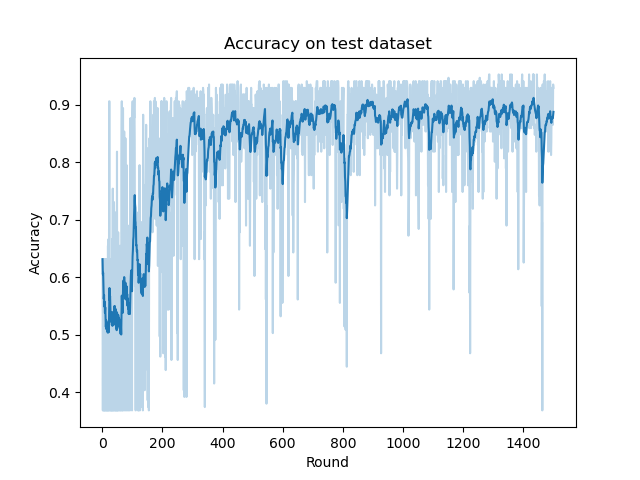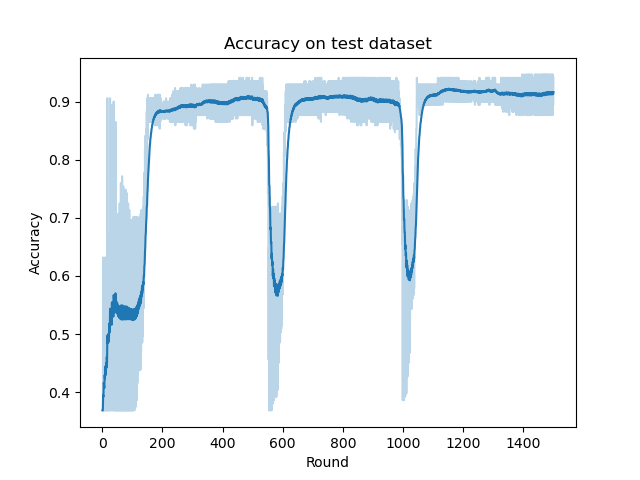## 1 分布式机器学习概述

1）对于计算量大的问题，分布式多机并行运算可以基本解决。不过需要与传统HPC中的共享内存式的多线程并行运算（如OpenMP）以及CPU-GPU计算架构做区分，这两种单机的计算模式我们一般称为计算并行

2）对于训练数据大的问题，需要将数据进行划分并分配到多个工作节点(Worker)上进行训练，这种技巧一般被称为数据并行。每个工作节点会根据局部数据训练出一个本地模型，并且会按照一定的规律和其他工作节点进行通信（通信内容主要是本地模型参数或者参数更新），以保证最终可以有效整合来自各个工作节点的训练结果并得到全局的机器学习模型。

3）对于模型规模大的问题，则需要对模型进行划分，并且分配到不同的工作节点上进行训练，这种技巧一般被称为模型并行。与数据并行不同，模型并行的框架下各个子模型之间的依赖关系非常强，因为某个子模型的输出可能是另外一个子模型的输入，如果不进行中间计算结果的通信，则无法完成整个模型训练。因此，一般而言，模型并行相比数据并行对通信的要求更高。

## 2 同步并行SGD算法描述与实现

SSGD对应的伪代码可以表述如下：from sklearn.datasets import load_breast_cancer
import numpy as np
from pyspark.sql import SparkSession
from sklearn.model_selection import train_test_split
from sklearn.metrics import accuracy_score
import matplotlib.pyplot as plt
import sys
import os

os.environ['PYSPARK_PYTHON'] = sys.executable

n_iterations = 1500  # Number of iterations
eta = 0.1
mini_batch_fraction = 0.1 # the fraction of mini batch sample
lam = 0 # coefficient of regular term

def logistic_f(x, w):
return 1 / (np.exp(-x.dot(w)) + 1)

""" Compute linear regression gradient for a matrix of data points
"""
y = point[-1]    # point label
x = point[:-1]   # point coordinate
# For each point (x, y), compute gradient function, then sum these up
return - (y - logistic_f(x, w)) * x

"""
assert(reg_type in ["none", "l2", "l1", "elastic_net"])
if reg_type == "none":
return 0
elif reg_type == "l2":
return w
elif reg_type == "l1":
return np.sign(w)
else:
return alpha * np.sign(w) + (1 - alpha) * w

def draw_acc_plot(accs, n_iterations):
def ewma_smooth(accs, alpha=0.9):
s_accs = np.zeros(n_iterations)
for idx, acc in enumerate(accs):
if idx == 0:
s_accs[idx] = acc
else:
s_accs[idx] = alpha * s_accs[idx-1] + (1 - alpha) * acc
return s_accs

s_accs = ewma_smooth(accs, alpha=0.9)
plt.plot(np.arange(1, n_iterations + 1), accs, color="C0", alpha=0.3)
plt.plot(np.arange(1, n_iterations + 1), s_accs, color="C0")
plt.title(label="Accuracy on test dataset")
plt.xlabel("Round")
plt.ylabel("Accuracy")
plt.savefig("ssgd_acc_plot.png")

if __name__ == "__main__":

D = X.shape
X_train, X_test, y_train, y_test = train_test_split(
X, y, test_size=0.3, random_state=0, shuffle=True)
n_train, n_test = X_train.shape, X_test.shape

spark = SparkSession\
.builder\
.appName("SSGD")\
.getOrCreate()

matrix = np.concatenate(
[X_train, np.ones((n_train, 1)), y_train.reshape(-1, 1)], axis=1)

points = spark.sparkContext.parallelize(matrix).cache()

# Initialize w to a random value
w = 2 * np.random.ranf(size=D + 1) - 1
print("Initial w: " + str(w))

accs = []
for t in range(n_iterations):
print("On iteration %d" % (t + 1))

(g, mini_batch_size) = points.sample(False, mini_batch_fraction, 42 + t)\
.treeAggregate(
(0.0, 0),\
seqOp=lambda res, g: (res + g, res + 1),\
combOp=lambda res_1, res_2: (res_1 + res_2, res_1 + res_2)
)

w -= eta * (g/mini_batch_size + lam * reg_gradient(w, "l2"))

y_pred = logistic_f(np.concatenate(
[X_test, np.ones((n_test, 1))], axis=1), w)
pred_label = np.where(y_pred < 0.5, 0, 1)
acc = accuracy_score(y_test, pred_label)
accs.append(acc)
print("iterations: %d, accuracy: %f" % (t, acc))

print("Final w: %s " % w)
print("Final acc: %f" % acc)

spark.stop()

draw_acc_plot(accs, n_iterations)

Initial w: [-0.36008689 -0.56946672 -0.111438   -0.69218272  0.14209017 -0.03984532
0.94087485  0.04861045 -0.61083642 -0.64141307  0.94030259  0.30646586
0.02675997  0.11238215 -0.86864834 -0.47662071 -0.0493882  -0.73569782
-0.92104136  0.91834097 -0.05942094  0.06995983 -0.3099812  -0.87308266
0.55421184  0.02251628  0.83765882 -0.69977596  0.02591093 -0.28081701
-0.21881969]

Final w: [ 3.58216967e+01  4.53599397e+01  2.07040135e+02  8.52414269e+01
4.33038042e-01 -2.93986236e-01  1.43286366e-01 -2.95961229e-01
-7.63362321e-02 -3.93180625e-01  8.19325971e-01  3.30881477e+00
-3.25867503e+00 -1.24769634e+02 -8.52691792e-01 -5.18037887e-01
-1.34380402e-01 -7.49316038e-01 -8.76722455e-01  9.23748261e-01
3.81531205e+01  5.56880612e+01  2.04895002e+02 -1.17586430e+02
8.92355523e-01 -9.40611324e-01 -9.24082612e-01 -1.16210791e+00
7.10117706e-01 -7.62921434e-02  4.48389687e+00]
Final acc: 0.929825

RDD.sample(withReplacement: bool, fraction: float, seed: Optional[int] = None) → pyspark.rdd.RDD[T]

$⩾0$；如果是无放回，参数fraction表示每个样本被采的概率，fraction必须要满足位于$\left[0,1\right]$区间内。

.treeAggregate(
(0.0, 0),\
seqOp=lambda res, g: (res + g, res + 1),\
combOp=lambda res_1, res_2: (res_1 + res_2, res_1 + res_2)
)

RDD.treeAggregate(zeroValue: U, seqOp: Callable[[U, T], U], combOp: Callable[[U, U], U], depth: int = 2) → U[source]

1. 对每个partition：

a. 初始化聚合结果为(0.0, 0)

b. 对当前partition的序列元素，依次执行聚合操作seqOp

c. 得到当前partition的聚合结果(partition_sum, partition_count)

1. 对所有partition：

a. 按照树行模式合并各partition的聚合结果，合并方法为combOp

b. 得到合并结果(total_sum, total_count)## 3 算法收敛性及复杂度分析

### 3.1 收敛性和计算复杂度## 参考

•  Zinkevich M, Weimer M, Li L, et al. Parallelized stochastic gradient descent[J]. Advances in neural information processing systems, 2010, 23.

•  刘浩洋，户将等. 最优化：建模、算法与理论[M]. 高教出版社, 2020.

•  刘铁岩，陈薇等. 分布式机器学习：算法、理论与实践[M]. 机械工业出版社, 2018.

__EOF__

• 本文作者： 猎户座
• 本文链接： https://www.cnblogs.com/orion-orion/p/16413182.html
• 关于博主： 本科CS系蒟蒻，机器学习半吊子，并行计算混子。
• 版权声明： 欢迎您对我的文章进行转载，但请务必保留原始出处哦(_^▽^*)。
• 声援博主： 如果您觉得文章对您有帮助，可以点击文章右下角推荐】一下。